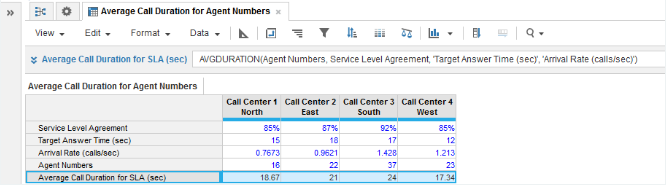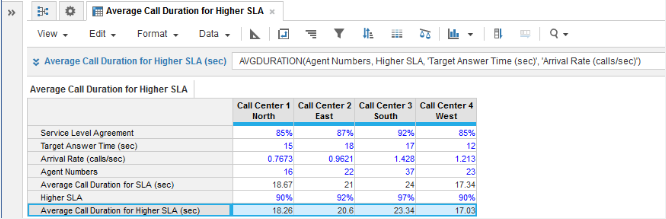1. Calculation functions
2. All Functions
3. Call Center Planning Functions
4. AVGDURATIONCalculates the average duration of calls that are answered while guaranteeing the service level agreement.

## Syntax

AVGDURATION(x, w, t, y)

where:

• x: number of servers, telephone lines, or agents
• w: service level agreement of the percentage of calls to be answered within the target answer time
• y: arrival rate of calls or the number of calls per unit of time

## Format

Input Format Output Format

x: number

w: number (percentage)

t: number (time unit)

y: number (time unit)

Number

## Arguments

The function uses the following arguments:

• x: Numeric line item, property, or expression
• w: Numeric line item, property, or expression
• t: Numeric line item, property, or expression - Must be the same time unit as used for argument y
• y: Numeric line item, property, or expression - Must be the same time unit as used for argument t

## Constraints

The function has the following constraints:

• All parameters are mandatory.
• All parameters must be a numeric line item, property, or expression.
• Time unit used for t and y parameters must be the same.
• Result line item must be numeric.
• Maximum number of agents is five million.

• None

## Example

Example 1

In this example, we have four call centers each covering a separate geographical area:

• Each call center must achieve a Service Level Agreement (SLA), expressed as the percentage of incoming calls that will be answered for a given target answer time.
• Agent Numbers is a numeric source line item for entering the agent staffing level at each call center.
• Target Answer Time (sec) is a numeric source line item for entering the target answer time for incoming calls.
• The arrival rates of calls are known for each call center from historical monitoring of operational performance and this baseline metric is entered in the Arrival Rate (calls/sec) numeric source line item.

We can now use AVGDURATION in the Average Call Duration for SLA (sec) numeric result line item to calculate the average duration of calls that can be maintained without compromising the agreed SLA:Example 2

In this second example, we use AVGDURATION to perform what-if analysis for average call duration. Suppose you want to determine what average call duration you can maintain for a higher SLA. The Higher SLA numeric source line item allows you to enter higher percentages for the SLA. The Average Call Duration for Higher SLA (sec) numeric result line item then provides the average call durations for the increased SLAs: